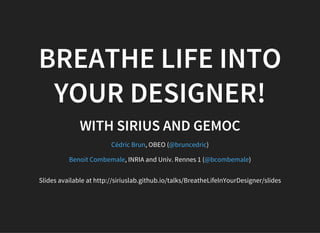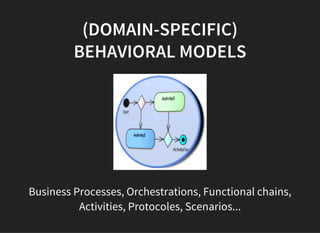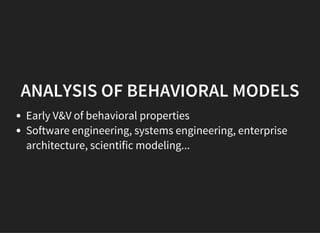Seu SlideShare está sendo baixado. ×

# Breathe life into your designer!

Anúncio
Anúncio
Anúncio
Anúncio
Anúncio
Anúncio
Anúncio
Anúncio
Anúncio
Anúncio
AnúncioCarregando em…3
×

1 de 30 Anúncio

# Breathe life into your designer!

You have your shiny new DSL up and running thanks to the Eclipse Modeling Technologies and you built a powerful tooling with graphical modelers, textual syntaxes or dedicated editors to support it. But how can you see what is going on when a model is executed ? Don't you need to simulate your design in some way ? Wouldn't you want to see your editors being animated directly within your modeling environment based on execution traces or simulator results?

You have your shiny new DSL up and running thanks to the Eclipse Modeling Technologies and you built a powerful tooling with graphical modelers, textual syntaxes or dedicated editors to support it. But how can you see what is going on when a model is executed ? Don't you need to simulate your design in some way ? Wouldn't you want to see your editors being animated directly within your modeling environment based on execution traces or simulator results?

Anúncio
Anúncio

### Breathe life into your designer!

1. 1. BREATHE LIFE INTO YOUR DESIGNER! WITH SIRIUS AND GEMOC , OBEO ( ) , INRIA and Univ. Rennes 1 ( ) Cédric Brun @bruncedric Benoit Combemale @bcombemale Slides available at http://siriuslab.github.io/talks/BreatheLifeInYourDesigner/slides
2. 2. (DOMAIN-SPECIFIC) BEHAVIORAL MODELS Business Processes, Orchestrations, Functional chains, Activities, Protocoles, Scenarios...
3. 3. ANALYSIS OF BEHAVIORAL MODELS Early V&V of behavioral properties Software engineering, systems engineering, enterprise architecture, scientific modeling...
4. 4. MODEL DEBUGGING Traversing step forward the states reached by the model through the application of the interpreter Intuitive model comprehension technique No abstraction gap Better turn-arounds ⇒ Fast convergence towards an acceptable design
5. 5. OMNISCIENT MODEL DEBUGGING Relies on an execution trace to revert the executed model into a prior state Forward/backward navigation into the execution trace No record & replay (similar) Replay
6. 6. OMNISCIENT DEBUGGING OF ACTIVITY DIAGRAMS SEE HTTP://GEMOC.ORG/TTC15
7. 7. REQUIRED TOOLS
8. 8. HOW TO GET YOUR OWN (DOMAIN-SPECIFIC) DEBUGGER?
9. 9. REQUIRED DEVELOPMENTS
10. 10. PROPOSED APPROACH: SIRIUS ANIMATION Xtend/Kermeta or Java to define the interpreter Sirius to define the animator by extension of the tooling description A generative approach for the trace manager A generic execution engine A generic control panel and timeline
11. 11. ANIMATING ARDUINO DESIGNER
12. 12. A SIMPLE ECLIPSE BASED TOOLING
13. 13. ARDUINO SIMULATOR 2 new plugins : fr.obeo.dsl.arduino.simulator fr.obeo.dsl.arduino.simulator.design                 - - - - - - - - - - - - - - - - - - - - - - - - - - - - - - - - - - - - - - - - - - - - - - - - - - - - - - - - - - - - L a n g u a g e f i l e s b l a n k c o m m e n t c o d e - - - - - - - - - - - - - - - - - - - - - - - - - - - - - - - - - - - - - - - - - - - - - - - - - - - - - - - - - - - - J a v a 1 4 2 2 9 3 1 8 1 1 8 5 X M L 5 0 0 3 2 9 M a v e n 2 1 2 1 8 5 4 - - - - - - - - - - - - - - - - - - - - - - - - - - - - - - - - - - - - - - - - - - - - - - - - - - - - - - - - - - - - S U M : 2 1 2 4 1 3 3 6 1 5 6 8 - - - - - - - - - - - - - - - - - - - - - - - - - - - - - - - - - - - - - - - - - - - - - - - - - - - - - - - - - - - -
14. 14. INTERPRETER               p u b l i c c l a s s L o o p S w i t c h e x t e n d s A r d u i n o S w i t c h { / / . . . @ O v e r r i d e p u b l i c O b j e c t c a s e S e n s o r ( S e n s o r s e n s o r ) { f i n a l P i n p i n = A r d u i n o U t i l s . g e t P i n ( s i m u l a t o r . g e t P r o j e c t ( ) , s e n s o r . g e t M o d u l e ( ) ) ; r e t u r n i n t e r p r e t O b j e c t V a l u e T o B o o l e a n ( s i m u l a t o r . g e t P i n L e v e l ( p i n ) } @ O v e r r i d e p u b l i c O b j e c t c a s e R e p e a t ( R e p e a t r e p e a t ) { f i n a l I n s t r u c t i o n f i r s t = r e p e a t . g e t I n s t r u c t i o n s ( ) . g e t ( 0 ) ; f o r ( i n t i = 0 ; i < r e p e a t . g e t I t e r a t i o n ( ) ; + + i ) { I n s t r u c t i o n c u r r e n t = f i r s t ; w h i l e ( c u r r e n t ! = n u l l ) { d o S w i t c h ( c u r r e n t ) ; c u r r e n t = c u r r e n t . g e t N e x t ( ) ; } } r e t u r n n u l l ; } / / . . . }
15. 15. EXECUTION ENGINE               p u b l i c c l a s s S i m u l a t o r D e b u g g e r e x t e n d s A b s t r a c t D S L D e b u g g e r { / / . . . p u b l i c v o i d s t a r t ( ) { n e w T h r e a d ( n e w R u n n a b l e ( ) { p u b l i c v o i d r u n ( ) { s p a w n R u n n i n g T h r e a d ( T h r e a d . c u r r e n t T h r e a d ( ) . g e t N a m e ( ) , s i m u l a t o r . g e t P r o j e c t ( ) ) ; s i m u l a t o r . i n i t ( ) ; s i m u l a t o r . l o o p ( ) ; } } , " A r d u i n o S i m u l a t o r " ) . s t a r t ( ) ; } p u b l i c v o i d u p d a t e D a t a ( S t r i n g t h r e a d N a m e , E O b j e c t i n s t r u c t i o n ) { i f ( ! h a r d w a r e F r a m e P u s h e d ) { p u s h S t a c k F r a m e ( T h r e a d . c u r r e n t T h r e a d ( ) . g e t N a m e ( ) , s i m u l a t o r . g e t P r o j e c t ( ) . g e t H a r d w a r e ( ) . g e t N a m e ( ) , s i m u l a t o r . g e t P r o j e c t ( ) . g e t H a r d w a r e ( ) , i n s t r u c t i o n ) ; h a r d w a r e F r a m e P u s h e d = t r u e ; } e l s e { s e t C u r r e n t I n s t r u c t i o n ( T h r e a d . c u r r e n t T h r e a d ( ) . g e t N a m e ( ) , i n s t r u c t i o n ) ; } f o r ( E n t r y < V a r i a b l e , O b j e c t > e n t r y : n e x t S u s p e n d V a r i a b l e s . e n t r y S e t ( ) ) { v a r i a b l e ( t h r e a d N a m e , s i m u l a t o r . g e t P r o j e c t ( ) . g e t H a r d w a r e ( ) . g e t N a m e ( ) , " v a r i a b l e " e n t r y . g e t K e y ( ) . g e t N a m e ( ) , e n t r y . g e t V a l u e ( ) , t r u e ) ; }
16. 16. ANIMATOR simulator.odesign customizing arduino.odesign
17. 17. ANIMATOR (2) Contributing actions Adapting style
18. 18. LEVERAGE THE SIRIUS ANIMATION RUNTIME Encapsulate step over/into/return in transactions Bridge the Eclipse Debug APIs and the EMF APIs Transmit events and requests Initialize the tooling extension Provide off-the-shelf ecore model for runtime data
19. 19. ECORE MODEL FOR RUNTIME DATA
20. 20. LAUNCH CONFIGURATIONS
21. 21. DEMO ... WITH NO CAT
22. 22. GEMOC The GEMOC ANR project ( ): A Language Workbench for concurrent execution and simulation of heterogeneous models The GEMOC Initiative ( ): GEMOC is an open international initiative that aims to coordinate and disseminate the research results regarding the support of the coordinated use of various modeling languages that will lead to the concept of globalization of modeling languages, that is, the use of multiple modeling languages to support coordinated development of diverse aspects of a system. http://gemoc.org/ins http://gemoc.org/
23. 23. GEMOC TECHNOLOGIES Ready for pilote project: Sirius Animation (animator designer and runtime, execution engine, trace manager) Ready for more R&D: MoccML and Timesquare for explicit concurrency specification in DSLs BCOoL for coordination patterns between DSLs
24. 24. INTRODUCING SIRIUS LAB
25. 25. technologies (EPL) which have proven helpful and are looking for an interest to bring it at full maturity level. Generic
26. 26. IN A NUTSHELL... POJO Animator (case of Arduino Designer) Code the Interpreter, the Execution Engine and possibly any additional tools quick results, easy to integrate, very few (or custom) execution control. Advanced Animator (case of Activity Diagram) Code the Interpreter and use the generic Execution Engine and Trace manager more analysis capabilities (concurrency) and rich execution control (panel, timeline...)
27. 27. TO BE CONTINUED... - 0.1.x - Read the - - to breath life into your designer Try executable Arduino Designer GEMOC Publications Join the GEMOC Initiative Get in touch with us